# High School Math : Understanding Angles in the Unit Circle

## Example Questions

### Example Question #15 : Graphing The Sine And Cosine Functions

In the unit circle, what is the angle in radians that corresponds to the point (0, -1)?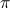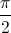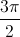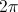Explanation:

On the unit circle, (0,-1) is the point that falls between the third and fourth quadrant.  This corresponds to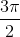.

### Example Question #1 : Understanding Angles In The Unit Circle

What is the reference angle for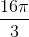?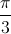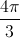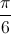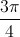To find the reference angle, subtract(1 trip around the unit circle) from the given angle until you reach an angle which is less than.